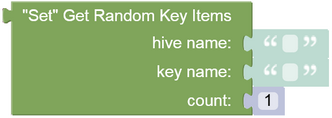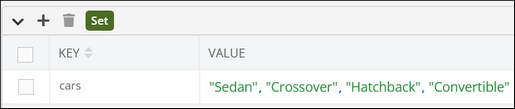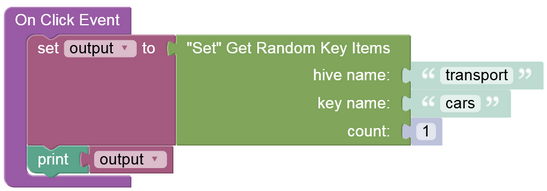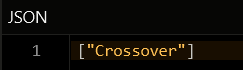# Get Random Values¶

### Description¶

This operation returns the specified number of random values from a set.

### Method¶

``````Backendless.Hive(hiveName).SetStore(keyName).getRandom(count): Promise<values[]>;
``````

where:

Argument                Description
`hiveName` Name of a hive where the operation is performed. String value.
`keyName` Key name identifying a set. String value.
`count` Optional parameter. Identifies the number of values to return in the response. Defaults to `1`. When the parameter value is a positive number, the  returned array contains only unique values. When the parameter value is negative, the returned array may include duplicate values.

### Return Value¶

An array containing values from the set. Each individual value is of any data type. The operation applies the following rules that impact the returned value:

• If the `count` parameter is not used, then this operation returns only one random value from the set.
• When a positive number is passed to the `count` parameter, then the returned array contains unique elements from the set.
• In case a negative number is passed to the `count` parameter, then the operation's behavior changes, and the returned array may contain duplicate elements.

### Examples¶

Return a Single Value

The example below returns a random value from the `'cars'` set.

``````await Backendless.Hive('transport').SetStore('cars').getRandom()
``````

where:

Argument                Description
`'transport'` Name of a hive where the operation is performed.
`'cars'` Key name identifying a set.

Return Multiple Unique Values

The example below returns five random values from the `'cars'` set. By specifying the `count` parameter you can change how many unique random values are returned.

``````await Backendless.Hive('transport').SetStore('cars').getRandom(5)
``````

where:

Argument                Description
`'transport'` Name of a hive where the operation is performed.
`'cars'` Key name identifying a set.

Get Multiple Values with Duplicates

The example below returns four random values from the `'cars'` set. Since the `count` parameter is a negative value, the response may include duplicate values.

``````await Backendless.Hive('transport').SetStore('cars').getRandom(-4)
``````

where:

Argument                Description
`'transport'` Name of a hive where the operation is performed.
`'cars'` Key name identifying a set.

You can find a sample response below demonstrating the presence of identical values.

``````[
"Sedan",
"Crossover",
"Crossover",
"Hatchback"
]
``````

### Codeless Reference¶where:

Argument                Description
`hive name` Name of a hive where the operation is performed.
`key name` Key name identifying a set.
`count` Number of random items to get.

This operation returns a list containing random values from the set. If the `count` parameter is a positive number, the returned list contains unique random values from the set. If the parameter is a negative number, the returned list may contain duplicates.

Consider the following Set storage:The example below retrieves a random value from the `"cars"` set:The logic produces the following output: